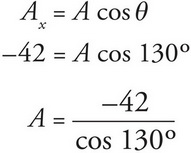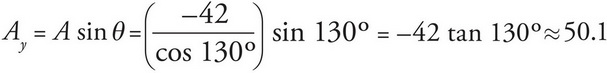# AP Physics 1 Question 189: Answer and Explanation

### Test Information

Question: 189

9. The x-component of vector A is -42, and the angle it makes with the positive x-direction is 130°. What is the y-component of vector A ?

• A. -65.3
• B. -50.1
• C. 50.1
• D. 65.3

Explanation:

C

The x-component of vector A can be used to calculate the magnitude of A:The y-component can then be calculated:which corresponds to (C).